Download Presentation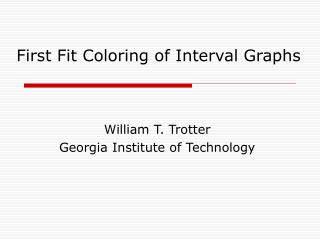First Fit Coloring of Interval Graphs

# First Fit Coloring of Interval Graphs - PowerPoint PPT Presentation

First Fit Coloring of Interval Graphs. William T. Trotter Georgia Institute of Technology. Interval Graphs. First Fit with Left End Point Order Provides Optimal Coloring. Interval Graphs are Perfect. Χ = ω = 4. What Happens with Another Order?. On-Line Coloring of Interval Graphs.I am the owner, or an agent authorized to act on behalf of the owner, of the copyrighted work described.
Download Presentation## First Fit Coloring of Interval Graphs

An Image/Link below is provided (as is) to download presentation

Download Policy: Content on the Website is provided to you AS IS for your information and personal use and may not be sold / licensed / shared on other websites without getting consent from its author.While downloading, if for some reason you are not able to download a presentation, the publisher may have deleted the file from their server.

- - - - - - - - - - - - - - - - - - - - - - - - - - E N D - - - - - - - - - - - - - - - - - - - - - - - - - -
Presentation Transcript### First Fit Coloring of Interval Graphs

William T. Trotter

Georgia Institute of Technology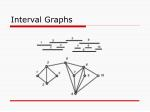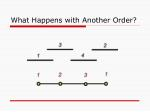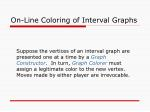On-Line Coloring of Interval Graphs

Suppose the vertices of an interval graph are presented one at a time by a Graph Constructor. In turn, Graph Colorer must assign a legitimate color to the new vertex. Moves made by either player are irrevocable.Optimal On-Line Coloring
• Theorem (Kierstead and Trotter, 1982)
• There is an on-line algorithm that will use at most 3k-2 colors on an interval graph G for which the maximum clique size is at most k.
• This result is best possible.
• The algorithm does not need to know the value of k in advance.
• The algorithm is not First Fit.
• First Fit does worse when k is large.How Well Does First Fit Do?
• For each positive integer k, let FF(k) denote the largest integer t for which First Fit can be forced to use t colors on an interval graph G for which the maximum clique size is at most k.
• Woodall (1976) FF(k) = O(k log k).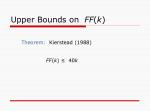Upper Bounds on FF(k)

FF(k) ≤ 40kUpper Bounds on FF(k)

Theorem: Kierstead and Qin (1996)

FF(k) ≤ 26.2kUpper Bounds on FF(k)

Theorem: Pemmaraju, Raman and

FF(k) ≤ 10kUpper Bounds on FF(k)

and Trotter (2003)

FF(k) ≤ 8kUpper Bounds on FF(k)

Theorem: Narayansamy and Babu(2004)

FF(k) ≤ 8k - 3Lower Bounds on FF(k)

Theorem: Kierstead and Trotter (1982)

There exists ε > 0 so that

FF(k) ≥ (3 + ε)k

when k is sufficiently large.Lower Bounds on FF(k)

Theorem: Chrobak and Slusarek (1988)

There exists ε > 0 so that

FF(k) ≥ 4k - 9

when k ≥ 4.Lower Bounds on FF(k)

Theorem: Chrobak and Slusarek (1990)

FF(k) ≥ 4.4 k

when k is sufficiently large.Lower Bounds on FF(k)

Theorem: Kierstead and Trotter (2004)

FF(k) ≥ 4.99 k

when k is sufficiently large.A Likely Theorem

Our proof that FF(k) ≥ 4.99 k is computer assisted. However, there is good reason to believe that we can actually write out a proof to show:

For every ε > 0, FF(k) ≥ (5 – ε) k when k is sufficiently large.A Negative Result and a Conjecture

However, we have been able to show that the Tree-Like walls used by all authors to date in proving lower bounds will not give a performance ratio larger than 5. As a result it is natural to conjecture that

As k tends to infinity, the ratio FF(k)/k tends to 5.